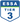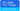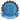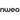# Distributive Property: 5 Clear Examples to Use in Class

All PostsWhat is the distributive property? Also known as the distributive law of multiplication, it’s one of the most commonly used properties in mathematics.

When you distribute something, you are dividing it into parts. In math, the distributive property helps simplify difficult problems because it breaks down expressions into the sum or difference of two numbers.

According to this principle, multiplying the total of two addends by a number will give us the exact same result as multiplying each addend individually by the number and then adding them together.

## Understanding distributive property

For expressions in the form of a(b+c), the distributive property shows us how to solve them by:

• Multiplying the number immediately outside parentheses with those insideWhat about PEMDAS? What happened to first evaluating what’s inside parentheses?

If your students are wondering why you aren’t following the order of operations you’ve taught them in the past, they’re not wrong.

However, when algebraic expressions have parentheses containing variables — a quantity that may change within the context of a mathematical problem, usually represented by a single letter — performing that operation isn’t possible.

## Distributive property of multiplication over addition

Regardless of whether you use the distributive property or follow the order of operations, you’ll arrive at the same answer. In the first example below, we simply evaluate the expression according to the order of operations, simplifying what was in parentheses first.Using the distributive law, we:

1. Multiply, or distribute, the outer term to the inner terms.
2. Combine like terms.
3. Solve the equation.

Let’s use a real-life scenario as an example of the distributive property.

Imagine one student and her two friends each have seven strawberries and four clementines. How many pieces of fruit do all three students have in total?

In their lunch bags — or, the parentheses — they each have 7 strawberries and 4 clementines. To know the total number of pieces of fruit, they need to multiply the whole thing by 3.

When you break it down, you’re multiplying 7 strawberries and 4 clementines by 3 students. So, you end up with 21 strawberries and 12 clementines, for a total of 33 pieces of fruit.## Distributive property of multiplication over subtraction

Similar to the operation above, performing the distributive property with subtraction follows the same rules — except you’re finding the difference instead of the sum.Note: It doesn’t matter if the operation is plus or minus. Keep whichever one is in the parentheses.

## Distributive property with variables

Remember what we said about algebraic expressions and variables? The distributive property allows us to simplify equations when dealing with unknown values.

Using the distributive law with variables involved, we can isolate x:

1. Multiply, or distribute, the outer term to the inner terms.
2. Combine like terms.
3. Arrange terms so constants and variables are on opposite sides of the equals sign.
4. Solve the equation and simplify, if needed.Note: When isolating variables (see step three) what you do to one side you must do to the other. In order to eliminate 12 on the left side, you must add twelve to both the left and right sides. The same goes for multiplication and division: to isolate x, divide each side by 4.

## Distributive property with exponents

An exponent is a shorthand notation indicating how many times a number is multiplied by itself. When parentheses and exponents are involved, using the distributive property can make simplifying the expression much easier.

1. Expand the equation.
2. Multiply (distribute) the first numbers of each set, outer numbers of each set, inner numbers of each set, and the last numbers of each set.
3. Combine like terms.
4. Solve the equation and simplify, if needed.Note: For step two, use the FOIL (first, outer, inner, last) technique to distribute each expression.

## Distributive property with fractions

Solving algebraic expressions with fractions looks more complicated than it is. Follow the steps outlined below to see how it’s done.

Hopefully, this step-by-step process helps your students understand how and why the distributive property can come in handy when simplifying fractions and complex numbers.

1. Identify the fractions. Using the distributive property, you’ll eventually turn them into integers.
2. For all fractions, find the lowest common multiple (LCM) — the smallest number that both denominators can fit neatly into. This will allow you to add fractions.
3. Multiply every term in the equation by the LCM.
4. Isolate variables adding or subtracting like terms on both sides of the equals sign.
5. Combine like terms.
6. Solve the equation and simplify, if needed.Note: In steps two and three, we find the LCM and use it to multiply the fractions in order to simplify and get rid of them. Need a quick refresher? See our blog post on how to multiply fractions.

## A variety of properties

Apart from distributive property, there are other commonly used properties such as the associative property and Commutative property.

Let's look at the associative property:

The associative property refers to grouping elements together. This rule states that how numbers (or whole numbers) are grouped within a math problem will not change the product.

a + (b +c) = (a + b) + c or 2 + (3 + 4) = (2 + 3) + 4

Example in multiplication:

5×4×2 = (5 x 4) x 2 = 20 x 2 = 40

This property works with multiplication, addition, subtraction, and division.

## Exploring distributive property in different ways

### 1. Prodigy

Prodigy is an adaptive, game-based learning math platform loved by more than one million teachers 150 million students around the world! It offers curriculum-aligned content from every major math topic in 1st to 8th grade, including how to:

• Use the distributive property to expand and solve expressions
• Fill in the missing numbers in equivalent expressions using the distributive propertyUsing Prodigy Math Game helps students learn and practice math beyond fact fluency and into DoK levels two and three. With questions like the one above, students will master the distributive property in no time at all enjoy our variety of guides and worksheets..

Interested in supplementing math lessons with an engaging game-based learning platform?

### 2. Word problems

The distributive property may not see applicable to everyday life, but let’s see it in action through some word problems!

Liam has diverse taste in music. Scrolling through the music on his phone, Liam’s friends find songs from three different genres: pop, metal, and country. There are six times as many metal songs as there are pop songs, and 11 times as many country songs as there are pop songs. If x represents the number of pop songs, what is the total number of songs Liam has on his phone? Write an expression. Simplify.

To get the number of metal songs, multiply the number of pop songs by five — 5x. To get the number of country songs, multiply the number of pop songs by 11 — 11x. Since you know x is the number of pop songs, you can write the expression as:The school’s soccer coach is providing his team with new uniforms: a jersey, a pair of shorts, and shin guards. One jersey costs \$15, one pair of shorts costs \$11, and a set of shin guards costs \$8.

How much does each uniform cost per team mate? Write an expression and simplify.How much will it cost in total if the team has 11 players? Write an expression and simplify.### 3. Arrays

Visual or hands-on manipulatives help students make sense of math and concretize abstract concepts. They’re especially helpful for deepening your students’ understanding of the distributive property.

Use objects, pictures, numbers — anything! — in rows and columns as a useful way to represent mathematical expressions like 4x5 and 5x9. Check out the example below on Indulgy:By breaking down expressions into bite-sized pieces, students can tackle larger and more challenging math problems. That’s where distributive property helps.

If a child has trouble answering 45, use smaller arrays and rewrite the expression as 4(3+2) or 4(3)+4(2). That’s four rows of three plus four rows of two, which is the same as an array of four rows of five.

## Final thoughts on the distributive property

As one of the most commonly used properties, it’s important to learn how to perform and apply the distributive property. Without it, clearing the parentheses wouldn’t be possible.

By incorporating EdTech resources, arrays, or math word problems, students should see the hands-on, practical applications of the distributive property.

Did one example work more effectively to engage students and deepen their understanding? There's only one way to find out — give them a try!

Prodigy Math Game is a game-based learning platform for math that’s easy to use for educators and students alike. Aligned with curricula across the English-speaking world, it’s used by over one million teachers and 150 million students!

••••